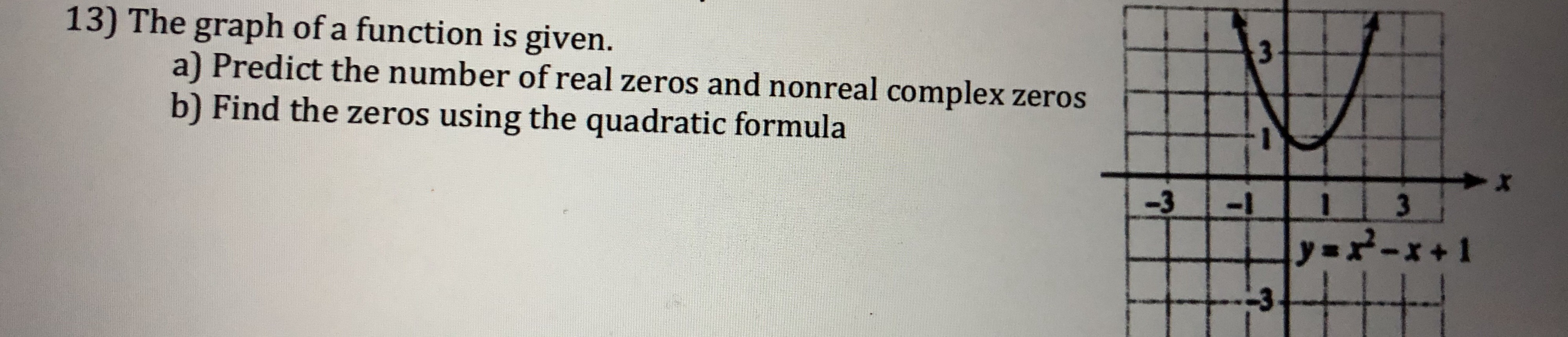# 13) The graph of a function is given.a) Predict the number of real zeros and nonreal complex zerosb) Find the zeros using the quadratic formula-313

Question
1 viewshelp_outlineImage Transcriptionclose13) The graph of a function is given. a) Predict the number of real zeros and nonreal complex zeros b) Find the zeros using the quadratic formula -313 fullscreen
check_circle

Step 1

Part (a)

We have been given a graph and we are required to predict the number of real and complex zeroes.

Since the given graph is for a parabola and it always lies above the x axis, therefore, it doesn't have any real solutions and it has 2 complex solutions.

Step 2

Part(b):

We have been given equation of the graph as y=x^2-x+1 and we are required to find its zeroes using quadradic formula. Please check below the quadratic formula and how the values o...

### Want to see the full answer?

See Solution

#### Want to see this answer and more?

Solutions are written by subject experts who are available 24/7. Questions are typically answered within 1 hour.*

See Solution
*Response times may vary by subject and question.
Tagged in

### Complex Numbers